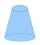# Cone A2V

Surface of cone in the plane is a circular arc with central angle of 126° and area 415 cm2.

Calculate the volume of a cone.

Result

V =  881.1 cm3

#### Solution:Leave us a comment of example and its solution (i.e. if it is still somewhat unclear...):Be the first to comment!#### To solve this example are needed these knowledge from mathematics:

Need help calculate sum, simplify or multiply fractions? Try our fraction calculator. Pythagorean theorem is the base for the right triangle calculator.

## Next similar examples:

1. Cut and coneCalculate the volume of the rotation cone which lateral surface is circle arc with radius 15 cm and central angle 63 degrees.
2. ConeCalculate volume and surface area of ​​the cone with diameter of the base d = 15 cm and side of cone with the base has angle 52°.
3. TowerHow many m2 of copper plate should be to replace roof of the tower conical shape with diameter 24 m and the angle at the vertex of the axial section is 144°?
4. Axial sectionAxial section of the cone is an equilateral triangle with area 208 dm2. Calculate the volume of the cone.
5. RotationRight triangle with legs 14 cm and 20 cm rotate around longer leg. Calculate the volume and surface area of the formed cone.
6. CanopyMr Peter has metal roof cone shape with a height of 127 cm and radius 130 cm over well. He needs paint the roof with anticorrosion. How many kg of color must he buy if the manufacturer specifies the consumption of 1 kg to 3.3 m2?
7. CapJesters hat is shaped a rotating cone. Calculate how much paper is needed to the cap 60 cm high when head circumference is 52 cm.
8. Rotary coneThe volume of the rotation of the cone is 472 cm3 and angle between the side of the cone and base angle is 70°. Calculate lateral surface area of this cone.
9. Lateral surface areaThe ratio of the area of the base of the rotary cone to its lateral surface area is 3: 5. Calculate the surface and volume of the cone, if its height v = 4 cm.
10. ConeCircular cone with height h = 29 dm and base radius r = 3 dm slice plane parallel to the base. Calculate distance of the cone vertex from this plane, if solids have the same volume.
11. 2x coneCircular cone height 84 cm was cut plane parallel with base. Volume of these two small cones is the same. Calculate the height of the smaller cone.
12. ConeCircular cone of height 15 cm and volume 5699 cm3 is at one-third of the height (measured from the bottom) cut by a plane parallel to the base. Calculate the radius and circumference of the circular cut.
13. SandpileAuto sprinkled with sand to an approximately conical shape. Workers wanted to determine the volume (amount of sand) and therefore measure the circumference of the base and the length of both sides of the cone (over the top). What is the volume of the san
14. Rotating coneCalculate volume of a rotating cone with base radius r=12 cm and height h=7 cm.
15. Truncated coneCalculate the height of the rotating truncated cone with volume V = 1115 cm3 and a base radii r1 = 7.9 cm and r2 = 9.7 cm.
16. Sphere in coneA sphere of radius 3 cm desribe cone with minimum volume. Determine cone dimensions.
17. Rotary coneRotary cone whose height is equal to the circumference of the base, has a volume 229 cm3. Calculate the radius of the base circle and height of the cone.